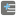• # Stalls out at check 11.

## Question related to mission_P Subsets Summing

I'm getting a fail after check 11 on this one.

It shows:

I'm assuming I'm failing the 12th check, but there's no message which states:

Here's my code.

```import itertools

def sum_list(l):
result =0
for x in range(len(l)):
result += sum(l[x])
return result

def make_comb (com_list):
combs = []
for i in range(1, len(com_list)+1):
l = [list(x) for x in itertools.combinations(com_list, i)]
combs.extend(l)

return combs

def checkio(data):

base_list = list(range(1,data+1))
change_list = base_list
combs =[]
final = 0

for i in reversed(range(1,data+1)):

combs  = make_comb(change_list)
final += sum_list(combs)
change_list.remove(i)

#print (combs)
return final
```